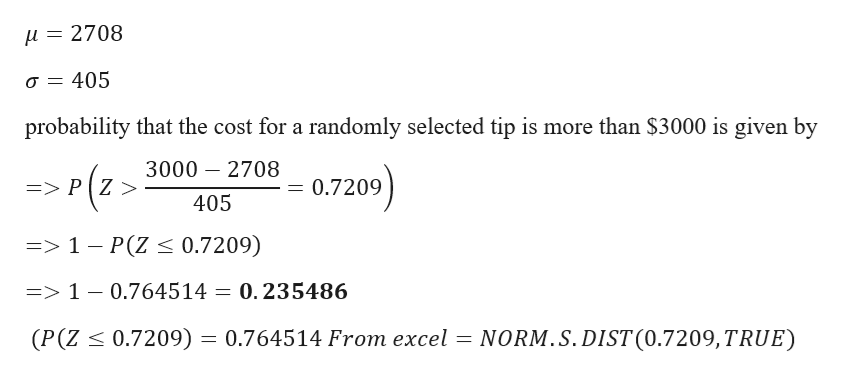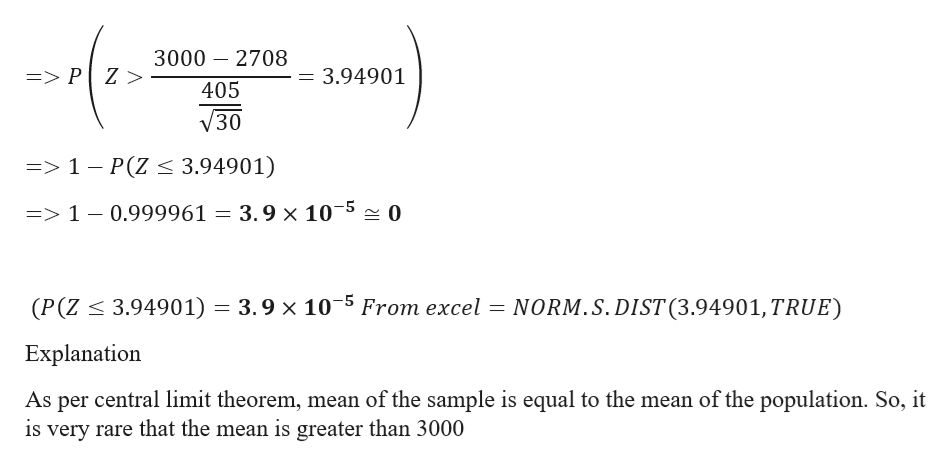# The average oversea tip cost is \$2708 per visitors. If we assume a normal distribution with a standard distribution of \$405, what is the probability that the cost for a randomly selected tip is more than \$3000? If we select a random sample of 30 oversea tips and find the mean of the sample, what is the probability that the mean is greater than \$3000? Explain?

Question

The average oversea tip cost is \$2708 per visitors. If we assume a normal distribution with a standard distribution of \$405, what is the probability that the cost for a randomly selected tip is more than \$3000? If we select a random sample of 30 oversea tips and find the mean of the sample, what is the probability that the mean is greater than \$3000? Explain?

check_circleExpert Solution
Step 1

Given Data

Mean = 2708

Standard deviation = 405help_outlineImage Transcriptionclosei = 2708 a 405 probability that the cost for a randomly selected tip is more than \$3000 is given by 3000 2708 => P Z > 0.7209 405 =>1 - P(Z < 0.7209) 0.235486 => 1 - 0.7645 14 NORM.S.DIST (0.7209,TRUE) (P (Z 0.7209) 0.764514 From excel fullscreen
Step 2

Mean of the sample would be same as the mean of the population as per central limit theorem

So mean = 2708

If we se...help_outlineImage Transcriptionclose3000 2708 => P Z > 3.94901 405 V30 => 1- P(Z < 3.94901) 3.9 x 1050 => 1 - 0.999961 (P(Z3.94901) 3.9 x 10 5 From excel = NORM.S. DIST (3.94901, TRUE) Explanation As per central limit theorem, mean of the sample is equal to the mean of the population. So, it is very rare that the mean is greater than 3000 fullscreen

### Want to see the full answer?

See Solution

#### Want to see this answer and more?

Solutions are written by subject experts who are available 24/7. Questions are typically answered within 1 hour*

See Solution
*Response times may vary by subject and question
Tagged in

### Other